Select Page

# CBSE Integrals Maths 12 Science Solutions for MCQ in English

CBSE Integrals Maths 12 Science Solutions for MCQ in English to enable students to get Solutions in a narrative video format for the specific question.

Expert Teacher provides CBSE Integrals Maths 12 Science Solutions for MCQ through Video Solutions in English language. This video solution will be useful for students to understand how to write an answer in exam in order to score more marks. This teacher uses a narrative style for a question from Integrals not only to explain the proper method of answering question, but deriving right answer too.

Please find the question below and view the Solution in a narrative video format.

Question:

Solution Video in English:

## Similar Questions from CBSE, 12th Science, Maths, Integrals

Question 1 :

Evaluate the following definite integral :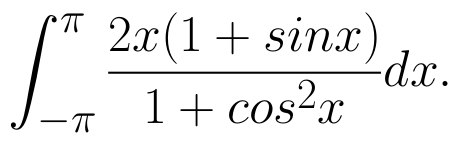(View Answer Video)

Question 2 : Evaluate :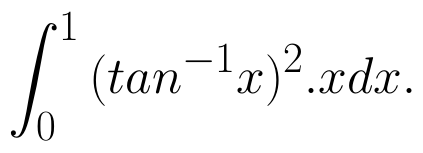(View Answer Video)

Question 3 : Evaluate: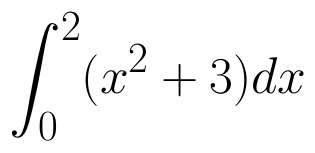as limit of sums.  (View Answer Video)

Question 4 : Write an antiderivative for the function,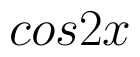using the method of inspection. (View Answer Video)

Question 5 : Write the value of :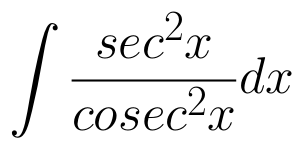(View Answer Video)

### Differential Equations

Question 1 : Write the differential equation representing the curve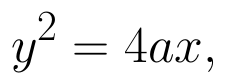where a is an arbitrary constant. (View Answer Video)

Question 2 : Find the particular solution of the differential equation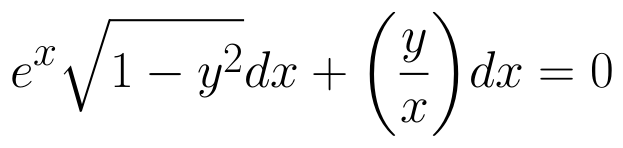given that y = 1 when x = 0. (View Answer Video)

Question 3 : Find the particular solution of the differential equation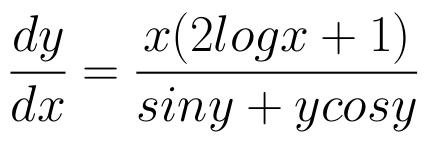given that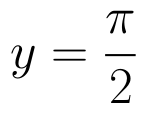where x = 1. (View Answer Video)

Question 4 : Solve the differential equation: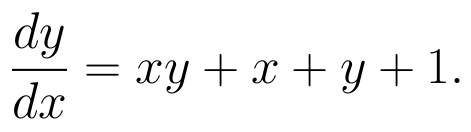(View Answer Video)

Question 5 : Find the particular solution of the differential equation :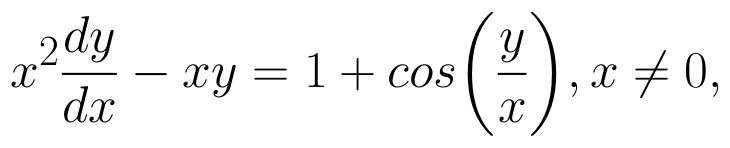when x = 1,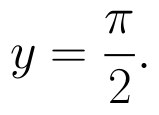(View Answer Video)

### Continuity and Differentiability

Question 1 : Findfor the function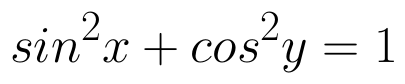. (View Answer Video)

Question 2 : Differentiate the function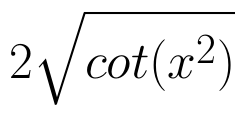with respect to x. (View Answer Video)

Question 3 : Differentiate w.r.t.x the function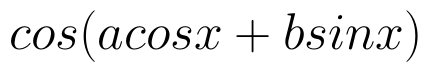, for some constant a and b. (View Answer Video)

Question 4 :  Find the second order derivative of the function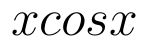. (View Answer Video)

Question 5 : Differentiate the function w.r.t.x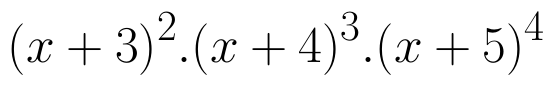. (View Answer Video)

### Application of Integrals

Question 1 : Find the area enclosed by the parabola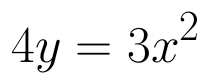and the line 2y = 3x + 12.   (View Answer Video)

Question 2 : Area lying in the first quadrant and bounded by the circle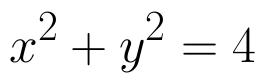and the lines x =0 and x = 2 is (View Answer Video)

Question 3 : Using integration, find the area of the region bounded by the curves: y = |x + 1| + 1, x = -3, x = 3, y = 0. (View Answer Video)

Question 4 : Using integration, find the area of the region bounded by the line 2x + y = 4, 3x - 2y = 6 and x - 3y + 5 = 0. (View Answer Video)

Question 5 : Find the area of smaller region bounded by the ellipse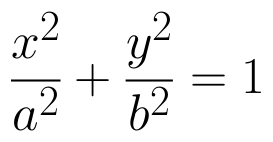and the line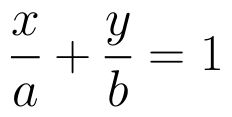. (View Answer Video)